• matlab提取矩阵非零元素
千次阅读
2021-04-18 05:51:20

MATLAB中求矩阵非零元的坐标：

方法1：

index=find(a);

[i,j]=ind2sub(size(a),index);

disp([i,j])

方法2：

[i,j]=find(a>0|a<0) %列出所有非零元的坐标

[i,j]=find(a==k) %找出等于k值的矩阵元素的坐标

所用函数简介：

IND2SUB Multiple subscripts from linear index.

IND2SUB is used to determine the equivalent subscript values

corresponding to a given single index into an array.

[I,J] = IND2SUB(SIZ,IND) returns the arrays I and J containing the

equivalent row and column subscripts corresponding to the index

matrix IND for a matrix of size SIZ.

For matrices, [I,J] = IND2SUB(SIZE(A),FIND(A>5)) returns the same

values as [I,J] = FIND(A>5).

[I1,I2,I3,...,In] = IND2SUB(SIZ,IND) returns N subscript arrays

I1,I2,..,In containing the equivalent N-D array subscripts

equivalent to IND for an array of size SIZ.

Class support for input IND:

float: double, single

FIND Find indices of nonzero elements.

I = FIND(X) returns the linear indices corresponding to

the nonzero entries of the array X. X may be a logical expression.

Use IND2SUB(SIZE(X),I) to calculate multiple subscripts from

the linear indices I.

I = FIND(X,K) returns at most the first K indices corresponding to

the nonzero entries of the array X. K must be a positive integer,

but can be of any numeric type.

I = FIND(X,K,'first') is the same as I = FIND(X,K).

I = FIND(X,K,'last') returns at most the last K indices corresponding

to the nonzero entries of the array X.

[I,J] = FIND(X,...) returns the row and column indices instead of

linear indices into X. This syntax is especially useful when working

with sparse matrices. If X is an N-dimensional array where N > 2, then

J is a linear index over the N-1 trailing dimensions of X.

[I,J,V] = FIND(X,...) also returns a vector V containing the values

that correspond to the row and column indices I and J.

Example:

A = magic(3)

find(A > 5)

finds the linear indices of the 4 entries of the matrix A that are

greater than 5.

[rows,cols,vals] = find(speye(5))

finds the row and column indices and nonzero values of the 5-by-5

sparse identity matrix.

codistributed/find

SUB2IND Linear index from multiple subscripts.

SUB2IND is used to determine the equivalent single index

corresponding to a given set of subscript values.

IND = SUB2IND(SIZ,I,J) returns the linear index equivalent to the

row and column subscripts in the arrays I and J for a matrix of

size SIZ.

IND = SUB2IND(SIZ,I1,I2,...,IN) returns the linear index

equivalent to the N subscripts in the arrays I1,I2,...,IN for an

array of size SIZ.

I1,I2,...,IN must have the same size, and IND will have the same size

as I1,I2,...,IN. For an array A, if IND = SUB2IND(SIZE(A),I1,...,IN)),

then A(IND(k))=A(I1(k),...,IN(k)) for all k.

Class support for inputs I,J:

float: double, single

更多相关内容
• matlab 如何把一个矩阵中的零数取出组成一行>... B=nonzeros(A)' B = 4 2 2 3 4 3 1 2 3 2matlab中怎么求数组中非零元素的个数matlab中求矩阵中非零元素的均值怎么求啊？ 代码： a(a==0)=NaN; mean(...

matlab 如何把一个矩阵中的非零数取出组成一行

>> A=randi([0 4],4) A = 4 2 1 0 2 3 2 3 0 4 0 2 0 3 0 0 >> B=nonzeros(A)' B = 4 2 2 3 4 3 1 2 3 2

matlab中怎么求数组中非零元素的个数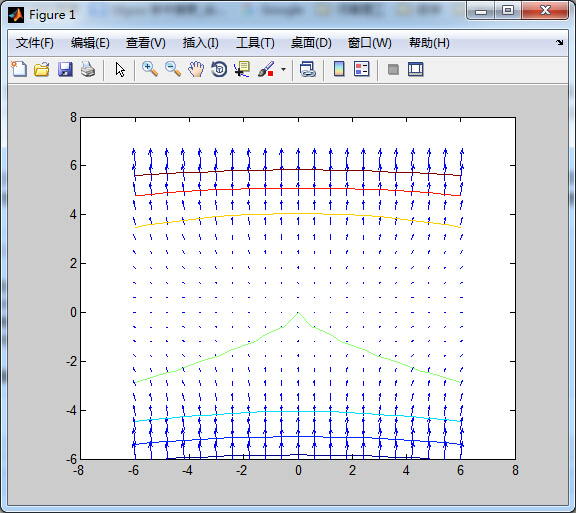matlab中求矩阵中非零元素的均值怎么求啊？ 代码： a(a==0)=NaN; mean(a,'omitnan') 把零替换成NaN，然后再用mean函数的'omitnan'参数计算均值，相比其他方法可以避免find函数或者判别式占用矩阵索引参数。比如一个多维矩阵A(x,y,z,)某一维的非零值计算均值，可用如上方法。

matlab中如何把一个矩阵中非零元素都变为1？

在Matlab中，空矩阵、零矩阵和全一矩阵是比较特殊的矩阵，它们在结构形式和矩阵元素的组织上有着独特的规律性。matlab中如何把一个矩阵中非零元素都变为1的方法是：

利用ones(N)生成一个N

Matlab如何提取非零元素

输入a=(x~=0)进行比较各个元素是否为0，如下图所示。

按回车键之后，可以看到x数组各个元素是否为0，为0就显示为0，不是就显示为1，如下图所示。

>> A = [-1 0 2;-3 -4 0;9 -78 1] A = -1 0 2 -3 -4 0 9 -78 1 >> A(A~=0) = 1 A = 1 0 1 1 1 0 1 1 1

MATLAB 中我想提取一列数据中所有非零的数并组成新比如一列数据[0,0,0,1,75,13,12,0,0,124,0,0,1216,87,3,6,3,0,0]; 想要假设原来的矩阵叫做A a=size(A,2); for i=1:a if A(i)==0 A(i)==[]; i=i-1; b=size(A,2); if i==b break end end end A 我猜的。

矩阵非空数据个数，matlab怎么统计？

矩阵NaN元素可用以下式子求得： num=numel(find(isnan(a)))； 因此非空数据个数： numel(a)-numel(find(isnan(a))) >> a a = 1 6 2 7 3 NaN 4 NaN 5 10 >> numel(a)-numel(find(isnan(a))) ans = 8 >>

小白求解： matlab 找出矩阵中的非零列

比如我现在有一个2*9的矩阵，A=, B=。现在我A=; B=; index = A&B; A(index) B(index) 望喜欢

在matlab里如何获得一个矩阵的行数或列数

在matlab里可以利用size函数获得一个矩阵的行数或列数。 size(a) 返回一个行向量，其元素包含 A 的相应维度的长度。例如，如果 a 是一个2×3矩阵，则 size(a) 返回向量 [2,3]。 具体说明如下。

第一步在matlab中命令行窗口中输入“a=[1 2 3;2 4

展开全文• 此代码提取子矩阵〜= 0 并创建新的矩阵组合子。 dataMat = 0 0 0 0 0 0 0 0 0 0 0 0 0 1 1 1 0 0 0 0 0 0 0 0 0 1 1 1 0 0 0 3 3 3 0 0 0 1 1 1 0 0 0 3 3 3 0 0 0 0 0 0 0 0 0 3 3 3 0 0 0 0 2 2 2 0 0 0 0 0 0 0 ...matlab
• 根据下标选取对应元素 1）简介 2）二维选取 3）多维选取 总结 前言 提示：这里可以添加本文要记录的大概内容： Matlab提供了功能丰富快速的矩阵计算方法，也有功能丰富的矩阵元素选取方法，由于循环计算的易理解和...

提示：文章写完后，目录可以自动生成，如何生成可参考右边的帮助文档

# 前言

提示：这里可以添加本文要记录的大概内容：

Matlab提供了功能丰富快速的矩阵计算方法，也有功能丰富的矩阵元素选取方法，由于循环计算的易理解和直观，新手通常会使用多层循环来选取矩阵元素，但是使用矩阵规则来选取其实会更快速和高效下面由简到繁介绍一下各种选取方法。

提示：以下是本篇文章正文内容，下面案例可供参考

## 1.简单切片

选取某行某列：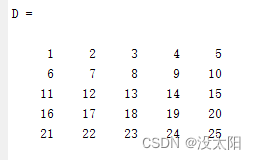D(2,:);
D(1:2,:);
D(:,2);
D(:,1:2);
D(2:3,1:2);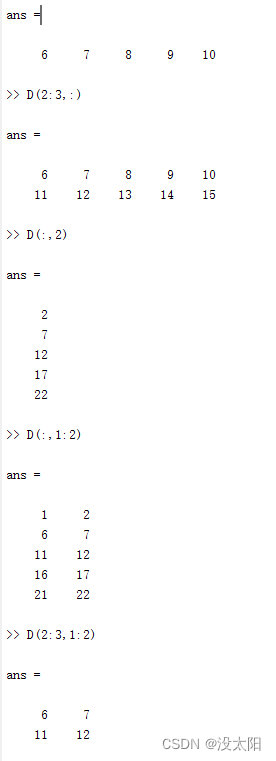## 2.根据条件选取

注意，当直接在变量后的括号内写入条件表达式时，返回的是符合条件的元素，这是根据逻辑表达式选取的，并被拉伸为一维向量。

D>10;
D(D>10);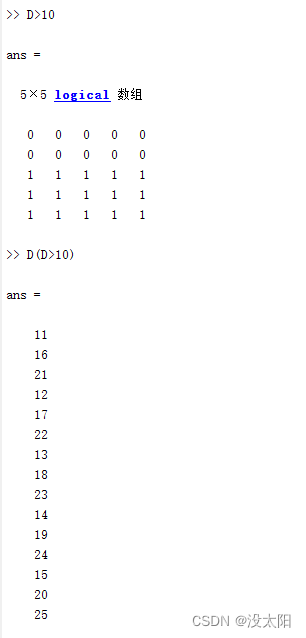## 3.根据下标选取对应元素

### 1）简介

当我们需要根据一个下标矩阵选取对应元素时，直接用对应行列返回的会是两个行向量的全部组合。

示例：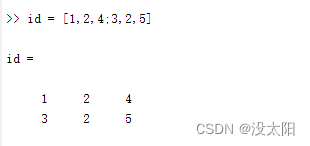当我们需要选择D矩阵中(1,3),(2,2),(4,5)位置的元素时，如果直接使用对应行向量选取：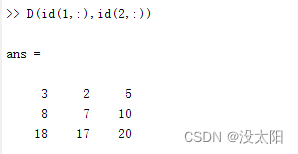会选取到(1,3),(1,2),(1,5),(2,3),…,(4,5)的所有组合，这时我们可以使用单下标的方法来选取对应元素；

在Matlab中，对于多维矩阵，除了使用直观上的D(1D,2D,3D,…,ND)的方法选取对应位置上的元素，也可以使用D(id)的方法来选取元素，比如对于18，在D中为4行3列，也可以表示为第14个元素。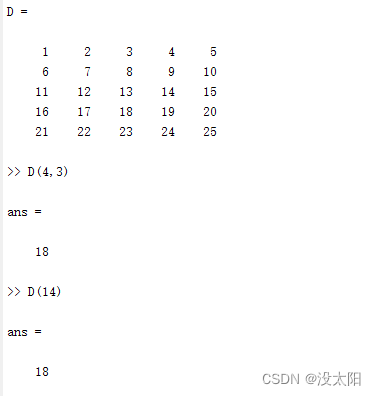### 2）二维选取

Matlab中维度是从后往前数的，二维中可以理解为先数列再数行：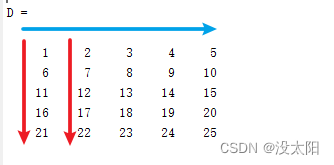也就是先数1，6，11，16，21;

因此第a行，第b列可以计算为第(d-1)*col+a，col为矩阵行数，也可以用size(D,1)获得，因此根据id矩阵选取对应元素可以写为：

D((id(2,:)-1)*size(D,1)+id(1,:))；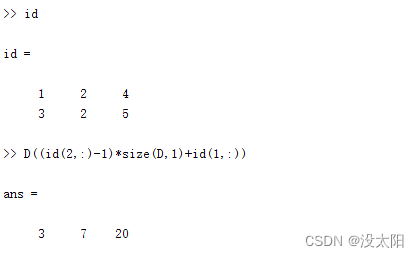### 3）多维选取

同理，对于多维，从后往前数即可，这里只演示到三维；

这里需要选择矩阵中的(1,2,1),(2,1,3),(1,1,3)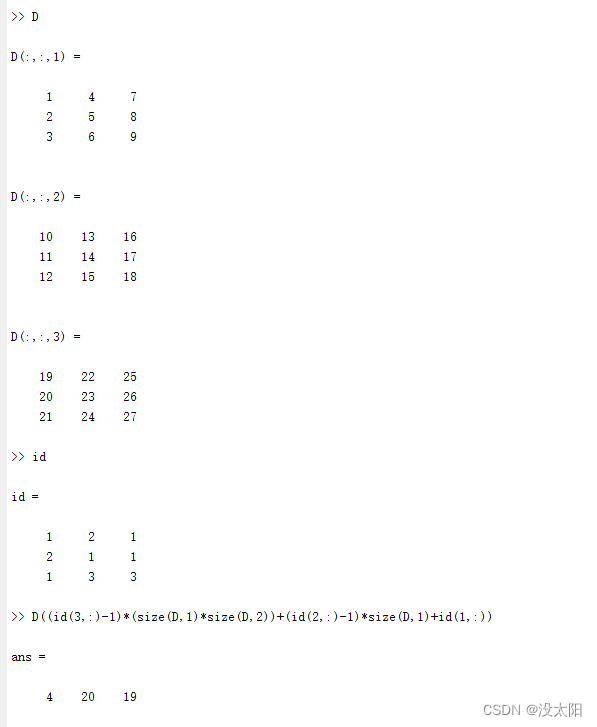当然，这里的id的计算也可以通过matlab函数sub2ind来替代。

# 总结

Matlab中，当遇到了对多维矩阵的元素选取因为使用多层循环嵌套极大增加运算时间可以试试将循环写为矩阵运算能够极大的提高运算速度。

展开全文matlab
• Matlab如何计算程序运行时间？Matlab如何提取矩阵前n行，或者后n行？Matlab如何检测矩阵中是否全为非零元素或否有非零元素

# 1.Matlab如何计算程序运行时间？

### 利用tic与toc组合计算程序运行时间，例：计算1+2+…+100的程序运行时间

tic
%% 代码块
sum=0;
for i=1:100
sum=sum+i;
end
%%
toc
disp(['运行时间: ',num2str(toc)]);# 2.Matlab如何提取矩阵前n行，或者后n行？

### eg：随机生成一个0~1之间的10×10的矩阵，提取矩阵的前4行和后4行

m=rand(10,10);
B=m(1:n,:); %提取矩阵前n行
C=m(end-n+1:end,:); %提取矩阵后n行


# 3.Matlab如何检测矩阵中是否全为非零元素或否有非零元素？

### eg：随机生成一个零矩阵，并利用all函数判断矩阵中是否全为非零元素；利用any检测矩阵中是否有非零元素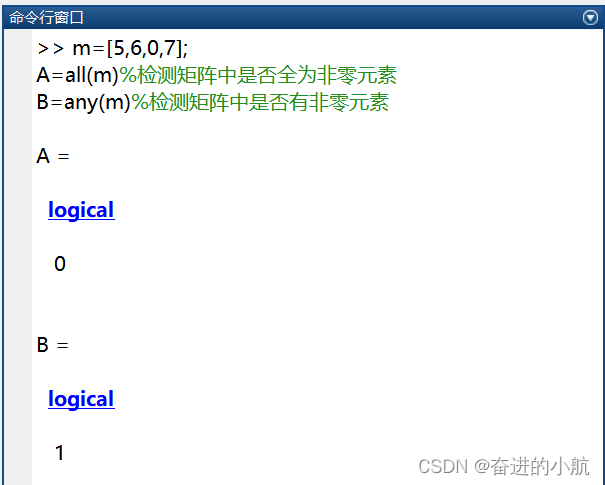展开全文matlab
• Matlab(12)——稀疏矩阵文章目录Matlab(12)——稀疏矩阵一、矩阵的...带状稀疏矩阵的稀疏存储方式①从带状稀疏矩阵提取对角线上元素②创建带状稀疏矩阵4.单位矩阵的稀疏存储方式一、矩阵的存储方式1.完全存储方式...
• a = [1 0 2 0 0 3 5 6]; b = a(a~=0);
• 非零的行cumsum是1 AND>非零的列cumsum是1 AND>元素本身不为.以下代码解决了这个问题：A = [0, 0, 3, 4;4, 3, 2, 0;2, 0, 2, 0];batches = cell(0);while any(A(:)~=0)selector = cumsum(A~=0, 1) .* cumsum...
• matlab中如何提取矩阵中指定位置元素 1）指定行和列:A(m,n),提取第m行，第n列 2）某一行全部元素：A(m,:) 3）某一列全部元素：A(:,n) 4) 取出某些行：A([m,n],: )（第m行和第n行组成一个矩阵） 5）取出某行到某行：A...
• 提取矩阵?某一行?或者?某一列?的方法 (2012-03-30 16:33:25) 转载 标签? matlab ? 提取 ? 一行 ? 一列 ? 矩阵 分类? Matlab 比如从一个6*6矩阵中提取它的第一行元素形成一个6维行向量 Ai行? Ai列 方法 Ai 提取矩阵A...
• MATLAB基础二 首先产生5阶两位随机整数矩阵A，再产生均值为0.6、方差为0.1的5阶正态分布随机矩阵B，最后验证(A+B)I=IA+BI（I为单位矩阵）。 rand函数：产生（0，1）开区间均匀分布的随机数x。 fix(a+(b-a+1)*x)...MATLAB 基础
• 现有矩阵A=[1 2 3;0,0,1;2,2,0]; [row,col]=find(A~=0); 或者[row,col]=find(A>0|A<0); 返回的row,col即为0坐标点的行和列。 row=[1;1;2;2;3;3]; col=[1;3;1;3;1;2].matlab
• 文章目录（1）取指定行和列的一个元素（2）取指定某一行全部元素（3）取指定某一列全部元素（4）取指定某些行的全部元素（输出一个矩阵）（5）取全部元素（按列拼接） （1）取指定行和列的一个元素 A(行，列) A(1,1)...matlab
• 使用matlab可以对矩阵很方便的操作，比如提取矩阵的部分与对其拼接，实现起来比较简单，其也可以用于图像矩阵的处理。 matlab中的使用方法如下： A=[1,2,3;4,5,6;7,8,9]; B=[1,1,1;2,2,2;3,3,3]; %提取矩阵A的1、2列...matlab
• 矩阵合并 / cell元素提取 / 修改矩阵内的某个元素matlab
• 矩阵行列和矩阵块的提取： 先随意设定一个6维的随机数矩阵矩阵a第2行 a(2,:) 取矩阵a第3列 a(:,3) 取矩阵a第2至第3列 取矩阵a第2至第3行 取矩阵2-3行和2-3列围出的矩阵矩阵的某元素 如a45 取矩阵第3列...matlab
• 关于矩阵索引的基础知识 索引相关知识在matlab常会出现，不熟悉常会致后续程序阅读出现误解 学习一段时间后，虽然有了框架基础，但是对该方面理解非常容易遇到阻碍， 遂决定做一篇总结 基本概念 冒号表达式 ...matlab 编程语言
• MATLAB学习笔记（2）MATLAB中的矩阵函数matlab 动态规划
• 输入------ A = 一个 nxm 矩阵（实数或虚数） 输出------- x = 存在于周边的矩阵元素的行向量，按顺时针方向取 A = An (n-2)x(m-2) 矩阵，与输入矩阵相同，外围不存在其元素（输入矩阵在函数内分解） 兼容性------...matlab
• ## MATLAB提取矩阵一部分

万次阅读 多人点赞 2020-01-10 11:53:55
格式A(m,n)，用于提取矩阵A中符合m,n要求的部分 1、提取某个元素，则m,n为数字标量，如A（2,3）为第二行第三列的元素。 2、提取某行某列 A(：，n)提取第n列的所有元素，如A(：，3)提取第三列的所有元素； A(m，...matlab
• MATLAB基本运算单元为矩阵，所以我们需要了解对矩阵的一些简单的语法操作。1、如何生成一个矩阵(来自百度)(1)元素输入法(2)设定步长生成形式为： A=a:inc:b可以看出a为初值，inc为步长，b为终值(界限) 相当于生成了...
• 1 3 >> x=[1,0,5,8] x = 1 0 5 8 >> y=find(x) y = 1 3 4 >> >> y1=2 y1 = 2 >> y2=9 y2 = 9 >> y3=-1 y3 = -1 >> if (y1>z) z=y1 elseif (y2>z) z=y2 else z=y3 end z = 2 >> 1.find函数用来寻找0元素的...
• 在矩阵中提取元素元素群 a = 1.0000 2.0000 3.0000 4.0000 5.0000 6.0000 7.0000 8.0000 9.0000 0 0 6.5000 5.0000 4.0000 3.0000 ...b=a([2,4],[1,3]) %提取矩阵a中2,4 两行的1,3 列数据 b = 4.00...matlab
• ## Matlab矩阵元素提取

千次阅读 2021-04-18 03:03:29
有一个1*41的矩阵矩阵的每个元素都是4*4的矩阵，如何提取4*4的矩阵中的特定元素？例如提取T(1)矩阵中的第一行第一列的元素。T(1) =1 0 0 0.40 -1 0 0.20 0 -1 00 0 0 1T(2)...
• 第2章 MATLAB矩阵及其运算 2.1 变量和数据操作 2.2 MATLAB矩阵 2.3 MATLAB运算 2.4 矩阵分析 2.5 矩阵的超越函数 2.6 字符串 2.7 结构数据和单元数据 2.8 稀疏矩阵 2.1 变量和数据操作 2.1.1 变量与赋值 1....
• Matlab矩阵元素与子矩阵的提取 Matlab矩阵元素与子矩阵的提取 >> %矩阵元素提取 >> A=[1 2 3;4 5 6;7 8 9;10 11 12] A = 1 2 3 4 5 6 7 8 9 10 11 12 >> A(3,1)%提取A矩阵第3行，第1列的元素 ...
• 对于零矩阵C[5*11]是5行11列. 使[1;2;3;4;5]行的 [3;4;6;8;9]列，五个元素为1如下代码： 基本思想：每列5个元素对应的列select_minQ-1乘以5，计算每列最后一个元素的坐标位置； #由于matlab没有index=0； select_...Matlab
• size()：获取矩阵的行数和列数(1)s=size(A),当只有一个输出参数时，返回一个行向量，该行向量的第一个元素矩阵的行数，第二个元素矩阵的列数。(2)[r,c]=size(A),当有两个输出参数时，size函数将矩阵的行数返回到...
•matlab...